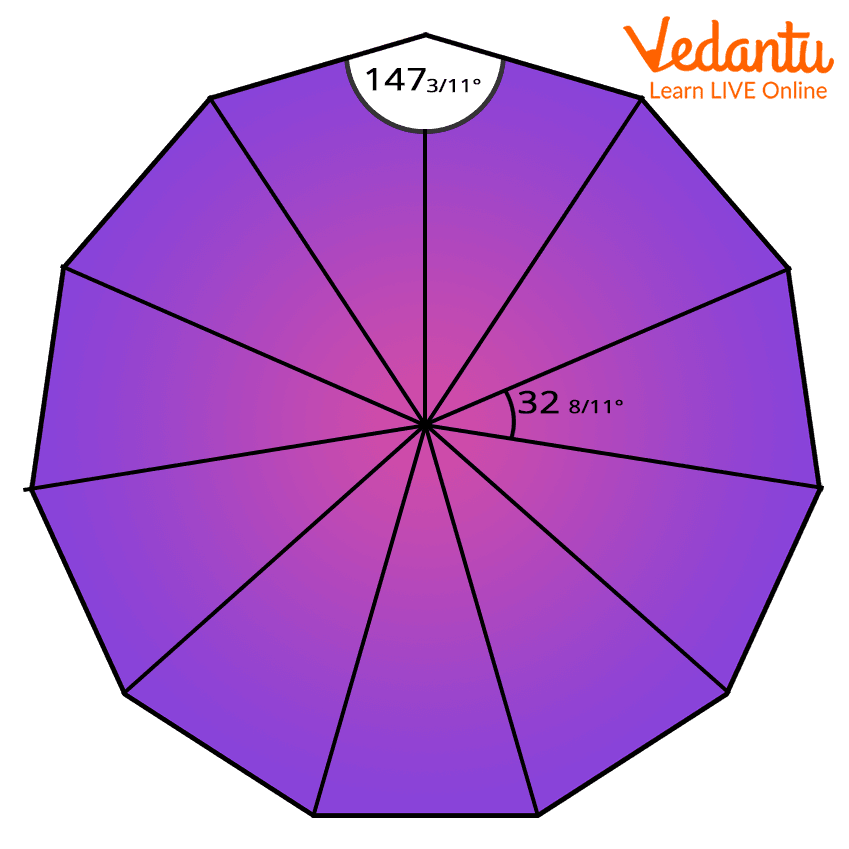Courses
Courses for Kids
Free study material
Offline Centres
More

# Hendecagon - Understanding Speciality of This ShapeLast updated date: 02nd Dec 2023
Total views: 154.8k
Views today: 3.54k## What is Hendecagon?

The word “Hendecagon” seems to be very familiar with the word “decagon”. A decagon is a shape that has ten sides and ten vertices whose internal angle measures 144 degrees each, so 10 sides mean 10 x 144 = 1440 degrees, which means the total measurement of all internal angles of a decagon is 1440 degrees.

We also call these shapes polygons (polygons are any closed curves with sets of lines joining each other, polygons can be triangles, quadrilaterals, and pentagons). So, a decagon is a 10-sided polygon. Now, what do we need to know about hendecagon? Well, a shape with 11-sides is called hendecagon and it has two synonyms, viz: undecagon or unidecagon.

Now, let us understand more about this shape starting with its properties and further information in detail.

## Properties of a Hendecagon

 Hendecagon Properties - Parameters Description Shape type 2-D What does a hendecagon look like?Hendecagon Type of polygon Regular Polygon Number of edges 11 Number of vertices 11 Interior angle measurement (each) $147\frac{3}{11}^{\circ}$  ≈ 147.273o Total sum of all internal angles 1620o

From the above text, we understand that a shape that has 10-sides is a decagon. When we talk about an 11-sided polygon, it becomes the hendecagon.

## Some Formulae of Hendecagon

Some of the basic formulae of hendecagon are as follows:

1. Perimeter of Hendecagon - $11\ast \sqrt{\frac{4\times Area\times tan\frac{\Pi }{4}}{11}}$

2. Perimeter of Hendecagon given circumradius - Perimeter = $22*Circumradius*sin(\frac{\pi}{11})$

3. Perimeter of Hendecagon given height - Perimeter = $(22*Height*tan(\frac{\pi/2}{11}))$

4. Area of Hendecagon given perimeter - Area = $(Perimeter)^2/(44*tan(\frac{\pi}{11}))$

5. Perimeter of Hendecagon given inradius - Perimeter = $22*Inradius*tan(\frac{\pi}{11})$

6. Area of Hendecagon - Area = $(\frac{11}{4})*(Side)^2*cot(\frac{\pi}{11})$

7. Perimeter of hendecagon given side = Perimeter = $11*Side$

## Know How to Pronounce ‘Hendecagon’

The phonetic spelling of hendecagon is ‘hen-dek-uh-gon’. If you are still facing problems in pronouncing the word then break the word into 4 syllables and try pronouncing it:

• ‘Hen’ first syllable

• ‘Dek’ second syllable

• ‘Uh’ third syllable

• ‘Gon’ fourth syllable

## Conclusion

The topic on hendecagon must have been fruitful for your child. The shape is not quite common, but knowing something uncommon is always amazing, isn’t it?

We have discussed the shape, talked about its properties, given the list of formulae of the hendecagon and also have explained how to pronounce the shape. Now we are going to share some frequently asked questions which will help your child to understand the shape well.

## FAQs on Hendecagon - Understanding Speciality of This Shape

1. How do you calculate the area of a hendecagon?

With the help of a definite formula we can calculate the area of a hendecagon.

The formula is =  $(\frac{11}{4})*(Side)^2*cot(\frac{\pi}{11})$ .

2. How many diagonals does a decagon have?

There are 44 diagonals in a decagon.

3. What is the other name for a hendecagon?

The other name for a hendecagon is 11-gon. This is because the shape has 11 sides, and thus the name 11-gon.